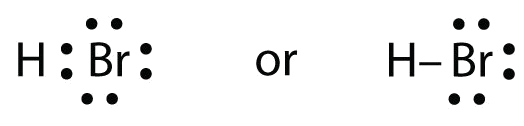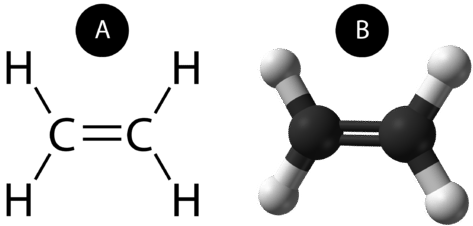# 3.3: Covalent Bonds

$$\newcommand{\vecs}{\overset { \rightharpoonup} {\mathbf{#1}} }$$ $$\newcommand{\vecd}{\overset{-\!-\!\rightharpoonup}{\vphantom{a}\smash {#1}}}$$$$\newcommand{\id}{\mathrm{id}}$$ $$\newcommand{\Span}{\mathrm{span}}$$ $$\newcommand{\kernel}{\mathrm{null}\,}$$ $$\newcommand{\range}{\mathrm{range}\,}$$ $$\newcommand{\RealPart}{\mathrm{Re}}$$ $$\newcommand{\ImaginaryPart}{\mathrm{Im}}$$ $$\newcommand{\Argument}{\mathrm{Arg}}$$ $$\newcommand{\norm}{\| #1 \|}$$ $$\newcommand{\inner}{\langle #1, #2 \rangle}$$ $$\newcommand{\Span}{\mathrm{span}}$$ $$\newcommand{\id}{\mathrm{id}}$$ $$\newcommand{\Span}{\mathrm{span}}$$ $$\newcommand{\kernel}{\mathrm{null}\,}$$ $$\newcommand{\range}{\mathrm{range}\,}$$ $$\newcommand{\RealPart}{\mathrm{Re}}$$ $$\newcommand{\ImaginaryPart}{\mathrm{Im}}$$ $$\newcommand{\Argument}{\mathrm{Arg}}$$ $$\newcommand{\norm}{\| #1 \|}$$ $$\newcommand{\inner}{\langle #1, #2 \rangle}$$ $$\newcommand{\Span}{\mathrm{span}}$$$$\newcommand{\AA}{\unicode[.8,0]{x212B}}$$

Learning Objectives

• Define covalent bond.
• Illustrate covalent bond formation with Lewis electron dot diagrams.

When valence electrons are shared between two atoms, they make a bond called a covalent bond. The driving force behind bond formation is stability - a lower potential energy state. There is a continuum of bonding between covalent bonds and ionic bonds (discussed in detail in Section 4.4) Let us illustrate a covalent bond by using H atoms, with the understanding that H atoms need only two electrons to fill the 1s subshell. Each H atom starts with a single electron in its valence shell:

$\mathbf{H\, \cdot }\; \; \; \; \; \mathbf{\cdot \: H}$

The two H atoms can share their electrons:

$\mathbf{H}\: \mathbf{: H}$

We can use circles to show that each H atom has two electrons around the nucleus, completely filling each atom’s valence shell:Because each H atom has a filled valence shell, this bond is stable, and we have made a diatomic hydrogen molecule. (This explains why hydrogen is one of the diatomic elements.) The shared pair of electrons is represented as a dash between the two atoms. It is possible for the same two atoms to share two pairs of electrons forming a double covalent bond or three pairs of electrons forming a triple covalent bond.

H–H

Because two atoms are sharing one pair of electrons, this covalent bond is called a single bond. As another example, consider fluorine. F atoms have seven electrons in their valence shell:These two atoms can do the same thing that the H atoms did; they share their unpaired electrons to make a covalent bond.Note that each F atom has a complete octet around it now:We can also write this using a dash to represent the shared electron pair:There are two different types of electrons in the fluorine diatomic molecule. The bonding electron pair makes the covalent bond. Each F atom has three other pairs of electrons that do not participate in the bonding; they are called lone pair electrons. Each F atom has one bonding pair and three lone pairs of electrons.

Covalent bonds can be made between different elements as well. One example is HF. Each atom starts out with an odd number of electrons in its valence shell:The two atoms can share their unpaired electrons to make a covalent bond:We note that the H atom has a full valence shell with two electrons, while the F atom has a complete octet of electrons.

Example $$\PageIndex{1}$$:

Use Lewis electron dot diagrams to illustrate the covalent bond formation in HBr.

Solution

HBr is very similar to HF, except that it has Br instead of F. The atoms are as follows:The two atoms can share their unpaired electron:Exercise $$\PageIndex{1}$$

Use Lewis electron dot diagrams to illustrate the covalent bond formation in Cl2.When working with covalent structures, it sometimes looks like you have leftover electrons. You apply the rules you learned so far and there are still some electrons hanging out there unattached. You can't just leave them there. So where do you put them?

## Multiple Covalent Bonds

Some molecules are not able to satisfy the octet rule by making only single covalent bonds between the atoms. Consider the compound ethene, which has a molecular formula of $$\ce{C_2H_4}$$. The carbon atoms are bonded together, with each carbon also being bonded to two hydrogen atoms.

two $$\ce{C}$$ atoms $$= 2 \times 4 = 8$$ valence electrons

four $$\ce{H}$$ atoms $$= 4 \times 1 = 4$$ valence electrons

total of 12 valence electrons in the molecule

If the Lewis electron dot structure was drawn with a single bond between the carbon atoms and with the octet rule followed, it would look like this:Figure $$\PageIndex{1}$$: Incorrect dot structure of ethene.

This Lewis structure is incorrect because it contains a total of 14 electrons. However, the Lewis structure can be changed by eliminating the lone pairs on the carbon atoms and having to share two pairs instead of only one pair.Figure $$\PageIndex{2}$$: Correct dot structure for ethene.

A double covalent bond is a covalent bond formed by atoms that share two pairs of electrons. The double covalent bond that occurs between the two carbon atoms in ethane can also be represented by a structural formula and with a molecular model as shown in the figure below.Figure $$\PageIndex{3}$$: (A) The structural model for $$\ce{C_2H_4}$$ consists of a double covalent bond between the two carbon atoms and single bonds to the hydrogen atoms. (B) Molecular model of $$\ce{C_2H_4}$$.

A triple covalent bond is a covalent bond formed by atoms that share three pairs of electrons. The element nitrogen is a gas that composes the majority of Earth's atmosphere. A nitrogen atom has five valence electrons, which can be shown as one pair and three single electrons. When combining with another nitrogen atom to form a diatomic molecule, the three single electrons on each atom combine to form three shared pairs of electrons.Figure $$\PageIndex{4}$$: Triple bond in $$\ce{N_2}$$.

Each nitrogen atom follows the octet rule with one lone pair of electrons and six electrons that are shared between the atoms.

## Summary

• Covalent bonds are formed when atoms share electrons.
• Lewis electron dot diagrams can be drawn to illustrate covalent bond formation.
• Double bonds or triple bonds between atoms may be necessary to properly illustrate the bonding in some molecules.